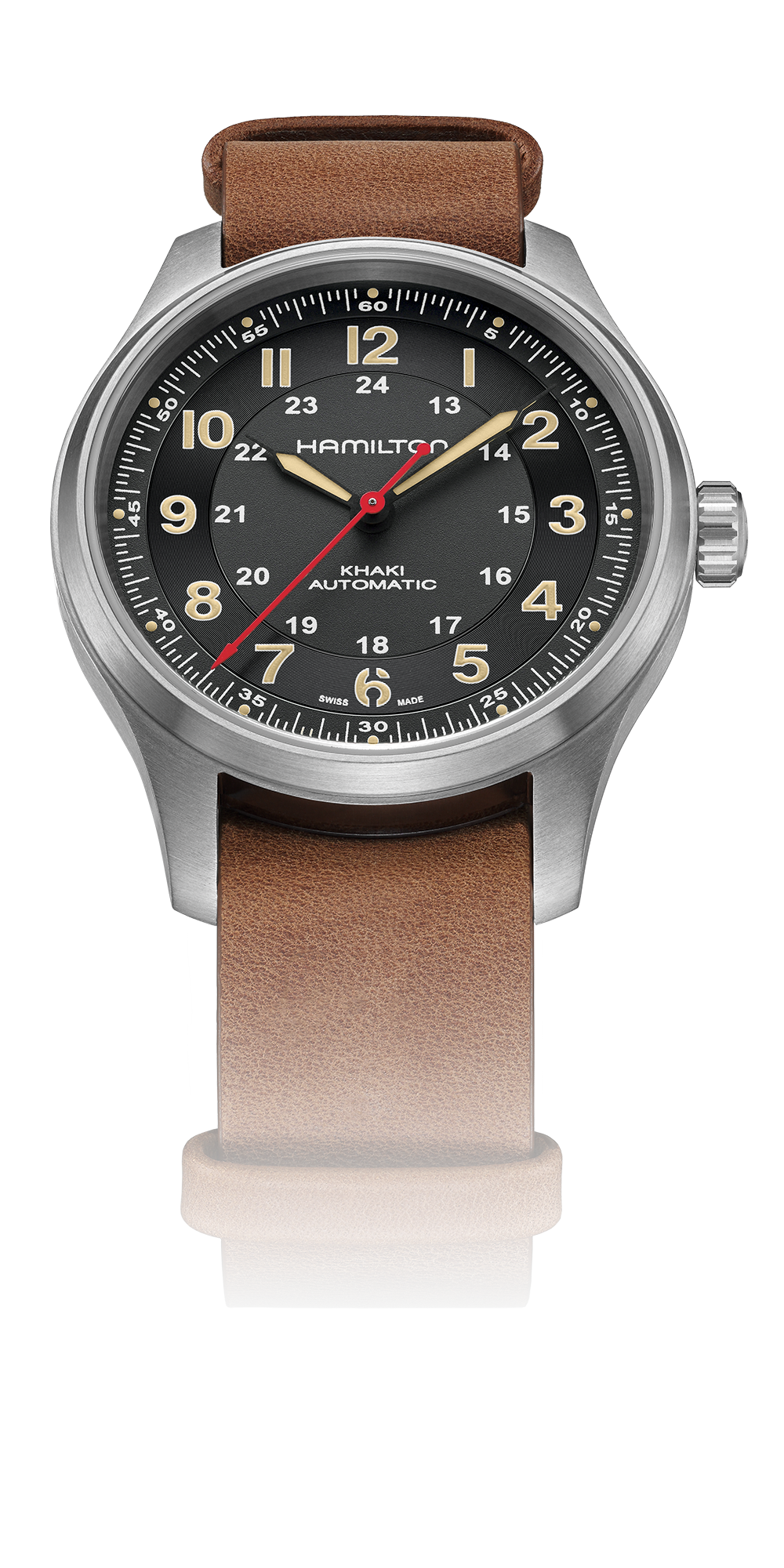# DANI ROJAS

<<<<<<<<<<<<<<<<<<<<<<<<

# 卡其野战系列钛合金自动腕表《孤岛惊魂®6》限量版

<<<<<<<<<<<<<<<<<<<<<<<<